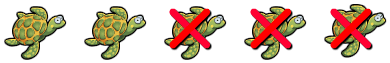# Subtracting Two Numbers Within 20

Rate 0 stars Common Core
Quiz size:
Message preview:
Someone you know has shared quiz with you:

To play this quiz, click on the link below:

https://www.turtlediary.com/quiz/subtracting-two-numbers-within-20.html

Hope you have a good experience with this site and recommend to your friends too.

Login to rate activities and track progress.
Login to rate activities and track progress.

Let's find the difference of 5 and 3.5 – 3 = ?

First, count the total number of turtles.There are five turtles.

Now count the number of turtles which are crossed.Out of 5 turtles, 3 are crossed.

Remaining number of turtles = 2

5 – 3 = 2

So, the difference of 5 and 3 is 2.

ds

A B C D E F G H I J K L M N O P Q R S T U V W X Y Z

### Help

##### Remember :

The smallest number is the one that comes first while counting.

##### Solution :

To arrange the given numbers in order from smallest to greatest, find the smallest number among all the given numbers.

21,27,23

21 is the smallest number.